### Home > MC2 > Chapter 2 > Lesson 2.3.2 > Problem2-110

2-110.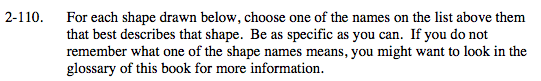obtuse triangle
rectangle
acute triangle

right triangle
equilateral triangle
rhombus

scalene triangle
parallelogram
trapezoid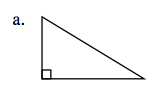What does the box marking mean?

Possible answers include a right or a scalene triangle.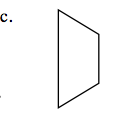Which names describe quadrilaterals (those with four sides)? Which of these have at least one pair of parallel sides?

Trapezoid.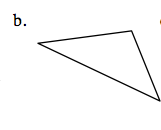Define an obtuse triangle, an acute triangle, and a scalene triangle. Which best describes this shape?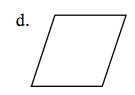What can you say about the side lengths?

Are the angles right angles?

Since the sides appear to have same lengths and the angles are not right angles, the best name for this shape is rhombus.